CAT  >  Test Level 1: Clocks & Calendars - 2

# Test Level 1: Clocks & Calendars - 2

Test Description

## 15 Questions MCQ Test Level-wise Tests for CAT | Test Level 1: Clocks & Calendars - 2

Test Level 1: Clocks & Calendars - 2 for CAT 2023 is part of Level-wise Tests for CAT preparation. The Test Level 1: Clocks & Calendars - 2 questions and answers have been prepared according to the CAT exam syllabus.The Test Level 1: Clocks & Calendars - 2 MCQs are made for CAT 2023 Exam. Find important definitions, questions, notes, meanings, examples, exercises, MCQs and online tests for Test Level 1: Clocks & Calendars - 2 below.
Solutions of Test Level 1: Clocks & Calendars - 2 questions in English are available as part of our Level-wise Tests for CAT for CAT & Test Level 1: Clocks & Calendars - 2 solutions in Hindi for Level-wise Tests for CAT course. Download more important topics, notes, lectures and mock test series for CAT Exam by signing up for free. Attempt Test Level 1: Clocks & Calendars - 2 | 15 questions in 40 minutes | Mock test for CAT preparation | Free important questions MCQ to study Level-wise Tests for CAT for CAT Exam | Download free PDF with solutions
 1 Crore+ students have signed up on EduRev. Have you?
Test Level 1: Clocks & Calendars - 2 - Question 1

### A watch was set right at 7 am. It gains 5 seconds in 3 minutes. In the afternoon of the same day, when the watch indicated 4:15, the true time was

Detailed Solution for Test Level 1: Clocks & Calendars - 2 - Question 1

Watch was set right at 7 am.
Time from 7 am to 4.15 pm = 9 hours 15 minutes = 37/7 hours
3 minutes 5 seconds of this clock = 3 minutes of the correct clock
Thus, 37/720 hours of this clock = 1/20 hours of the correct clock
Therefore, 37/4 hours of this clock =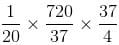hours of the correct clock
= 9 hours of the correct clock
Therefore, 9 hours 15 minutes of this clock = 9 hours of the correct clock
Hence, the correct time is 9 hours after 7 am i.e. 4 pm.

Test Level 1: Clocks & Calendars - 2 - Question 2

### A clock is so placed that at 12 noon, its minute hand points towards Northeast. In which of the following directions does its hour hand point at 1.30 pm?

Detailed Solution for Test Level 1: Clocks & Calendars - 2 - Question 2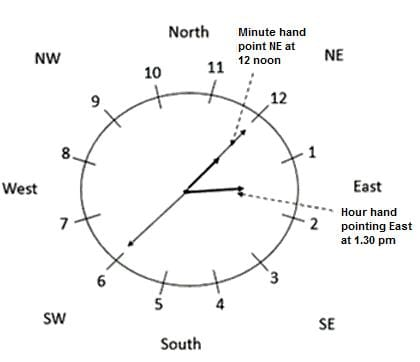Clearly, Hour hand will point in East direction at 1.30 pm.

Test Level 1: Clocks & Calendars - 2 - Question 3

### What time will it be 18 hours from now in India, if in USA, it is 4 hours 15 minutes ? (Given that India is 9 hours 30 minutes ahead of USA)

Detailed Solution for Test Level 1: Clocks & Calendars - 2 - Question 3

We know that the time difference between India and USA is 9 hours 30 minutes.
India is 9 hours 30 minutes  ahead of USA.
Time in India = 4 hours 15 minutes + 9 hours 30 minutes = 13 hours 45 minutes
Required time after 18 hrs = 13 hours 45 minutes + 18 hrs = 7 hours 45 minutes
Hence, it is 7 hours 45 minutes  the next morning.

Test Level 1: Clocks & Calendars - 2 - Question 4

At what angle are the hands of a clock inclined at 20 minutes past 6 a.m.?

Detailed Solution for Test Level 1: Clocks & Calendars - 2 - Question 4

Apply direct formula to calculate the angle:
30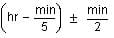∴ Required angle = 30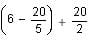(Take +ve because the hour hand is ahead of the minute hand.)
= 60 + 10 = 70°

Test Level 1: Clocks & Calendars - 2 - Question 5

At what angle are the hands of a clock inclined at 15 minutes past 5?

Detailed Solution for Test Level 1: Clocks & Calendars - 2 - Question 5

Apply direct formula: 30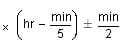Thus, required angle = 30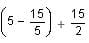(Take +ve sign because the hour hand is ahead of the minute hand.)
Thus, required time = 60 + 7.5 = 67.5°

Test Level 1: Clocks & Calendars - 2 - Question 6

Which of the following years has the same calendar as 1700?

Detailed Solution for Test Level 1: Clocks & Calendars - 2 - Question 6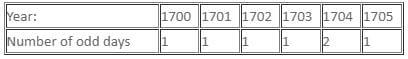Total number of odd days from 1700 to 1705 is remainder (7/7) i.e. 0.
So, after the year 1705, the year i.e 1706 has the same calendar as 1700.
Therefore, option c is the correct answer.

Test Level 1: Clocks & Calendars - 2 - Question 7

At what time between 5 and 6 are the hands of a clock coincident?

Detailed Solution for Test Level 1: Clocks & Calendars - 2 - Question 7

Hands of the clock will be coincident after,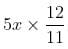minutes past x, where x is the any whole number from 1 to 12.
∴ Between 5 and 6, the hands will be coincident after, 5 × 5 ×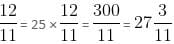minutes past 5

Test Level 1: Clocks & Calendars - 2 - Question 8

Rocky went out between 7 o'clock and 8 o'clock. At 8:30 p.m., he realised that the minute hand of the clock was as much behind the hour hand as the hour hand was behind the minute hand when he had gone out. At what time after he went out did Rocky look at his watch?

Detailed Solution for Test Level 1: Clocks & Calendars - 2 - Question 8

At 8:30 p.m., angular distance between hour hand and minute hand is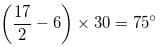When Rocky went between 7 to 8 p.m., the angular distance was 75 degrees.
At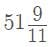minutes past 7 o'clock, angle made by minute hand =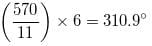Angle made by hour hand =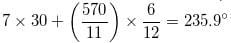Difference = 310.9 - 235.9 = 75°
Hence, option (a) is the correct option.

Test Level 1: Clocks & Calendars - 2 - Question 9

If the hands of a clock coincide every 66 minutes (true time), how much does the clock gain or lose every hour?

Detailed Solution for Test Level 1: Clocks & Calendars - 2 - Question 9

In a correct clock, the minute hand overlaps the hour hand every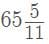minutes.
∴ Gain in 66 minutes =- 66 = - 6/11 minutes. Negative gain is a loss.
∴ Loss in 1 hour =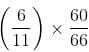minutes = 60/121 minutes

Test Level 1: Clocks & Calendars - 2 - Question 10

At what time between 4:15 a.m. and 5:05 a.m. will the angle between the hour hand and the minute hand of a clock be the same as the angle between the hands at 8:45 p.m?

Detailed Solution for Test Level 1: Clocks & Calendars - 2 - Question 10

At 4 o'clock, the angle between the hands is 120 degrees.
At 8:45 p.m, the angle is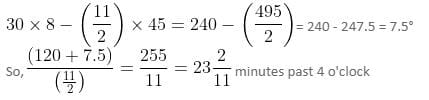Test Level 1: Clocks & Calendars - 2 - Question 11

If the hour-hand and the minute-hand of a clock coincide every 64 minutes, how much does the clock gain or lose everyday?

Detailed Solution for Test Level 1: Clocks & Calendars - 2 - Question 11

55 min spaces are covered in 60 min
60 min. spaces are covered in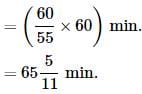Loss in 64 min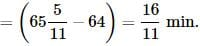Loss in 24 hrs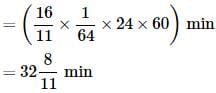Test Level 1: Clocks & Calendars - 2 - Question 12

At what time will the hands of a clock be together between 3 o'clock and 4 o'clock?

Detailed Solution for Test Level 1: Clocks & Calendars - 2 - Question 12

Using direct formula,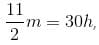where m - minutes and h - hours
Time, between 3 o'clock and 4 o'clock, at which the hands will be together =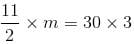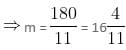minutes past 3 o'clock

Test Level 1: Clocks & Calendars - 2 - Question 13

A defective watch which gains uniformly, is 5 minutes slow at 8 o'clock in the morning on Sunday and it is 5 minutes 48 seconds fast at 8 pm on following Sunday. When was it correct?

Detailed Solution for Test Level 1: Clocks & Calendars - 2 - Question 13

Sunday 8 am to next Sunday 8 pm time duration 24 × 7 + 12 = 180 hrs.
In 180 hrs total gain = 10 min 48 s = 648 s
Gain of 5 min = 300 s will be obtained in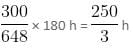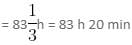Hence, Sunday 8 am + 83 h 20 min = Wednesday 7 : 20 p.m.

Test Level 1: Clocks & Calendars - 2 - Question 14

How many times are the hands of a clock at right angles in a day?

Detailed Solution for Test Level 1: Clocks & Calendars - 2 - Question 14

Hands will be at right angle twice every hour except from 2 to 4 and 8 to 10 where hands will be at right angle only thrice instead of four times.
Thus, hands will be at right angle 22 times in 12 hours or 44 times in 24 hrs.

Test Level 1: Clocks & Calendars - 2 - Question 15

At what time between 4 and 5 will the hands of a watch point in the opposite directions?

Detailed Solution for Test Level 1: Clocks & Calendars - 2 - Question 15

Hands are pointing in opposite directions. It means hands are 180° apart or they are 30 minutes apart.
Required time = (5x + 30) × 12/11 minutes past x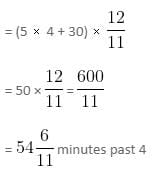## Level-wise Tests for CAT

5 docs|272 tests
 Use Code STAYHOME200 and get INR 200 additional OFF Use Coupon Code
Information about Test Level 1: Clocks & Calendars - 2 Page
In this test you can find the Exam questions for Test Level 1: Clocks & Calendars - 2 solved & explained in the simplest way possible. Besides giving Questions and answers for Test Level 1: Clocks & Calendars - 2, EduRev gives you an ample number of Online tests for practice

## Level-wise Tests for CAT

5 docs|272 tests

### How to Prepare for CAT

Read our guide to prepare for CAT which is created by Toppers & the best Teachers